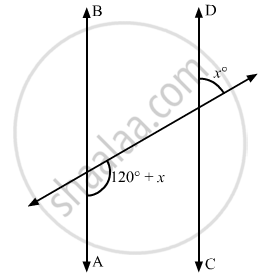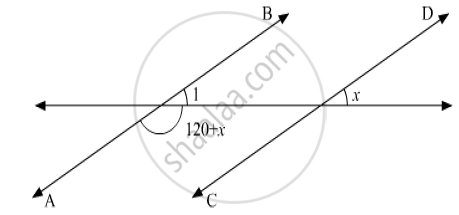# In the Given Figure, If Ab || Cd, Then the Value of X is - Mathematics

MCQ

In the given figure, if AB || CD, then the value of x is• 20°

• 30°

• 45°

• 60°

#### Solution

Here AB || CDAlso, ∠1 and x are the two corresponding angles.

Then, according to the Corresponding Angles Axiom, which states:

If a transversal intersects two parallel lines, then each pair of corresponding angles are equal.

Therefore,

∠1  = x

Also, ∠1 and  (120° + x) form a linear pair, therefore, their sum must be supplementary.

Therefore,

∠1 + (120° + x) = 180°

On substituting ∠1 = x in equation above, we get:

x + 120° + x = 180°

2x + 120° = 180°

2x = 180° - 120°

2x = 60°

x = (60°)/2

x = 30°

Concept: Parallel Lines and a Transversal
Is there an error in this question or solution?

#### APPEARS IN

RD Sharma Mathematics for Class 9
Chapter 10 Lines and Angles
Q 11 | Page 53